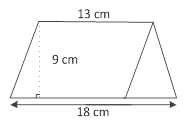Sample Problem

The figure is made up of a parallelogram and a triangle. Find its area.Its area is cm2.

Solution

Base of the parallelogram

= 13 cm

Height of the parallelogram

= 9 cm

Area of the parallelogram

= 13 cm × 9 cm

= 117 cm2

Base of the triangle

= 18 cm − 13 cm

= 5 cm

Height of the triangle

= 9 cm

Area of the triangle

= 1/2 × (5 cm × 9 cm)

= 22.5 cm2

∴ 117 cm2 + 22.5 cm2 = 139.5 cm2

Its area is 139.5 cm2.